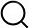# Also in the Article

Statistical Analysis

Procedure

Statistical analysis was performed using SPSS version 25.0 for Windows (SPSS Inc., IBM, Armonk, NY, United States). Data were tested for normal distribution (Shapiro-Wilk Test) and homogeneity of variance (Levene-Test for equality of variances). Mean differences of anthropometric and performance parameters between groups (normal weight vs. overweight vs. obese) were tested using a one-way analysis of variance (ANOVA) (Bortz, 1999). Differences between means were considered meaningful if p < 0.05 and partial eta-squared (ηp2) > 0.15 (Richardson, 2011). Alpha level is reported as exact p values and was not set dichotomously as significant or non-significant as recommended by Hurlbert et al. (2019). Post hoc pairwise comparisons were conducted using Bonferroni corrected T-tests. Due to the relatively small number of cases in each age group (n = 11) and in order to avoid an overestimation of mean differences, interpretation of results was primarily based on ηp2. For clarity, magnitude of group differences were interpreted using the following criteria: 0.02, a small difference; 0.13, a moderate difference; 0.26, a large difference. The effect size (d) (the mean difference of scores divided by the pooled SD) was calculated for each parameter (Hartmann et al., 1992), as is interpreted as trivial (<0.20), small (≥0.20–0.49), moderate (≥0.50–0.79), and large (≥0.80). Pearson’s product moment correlations were calculated and used to determine the relationship between anthropometric and performance (physical and academic) parameters. A magnitude of correlation (r) between the measures of <0.1, 0.1–0.3, 0.3–0.5, 0.5–0.7, and 0.7–0.9, >0.9, were considered trivial, small, moderate, large, very large, and almost perfect, respectively.

Note: The content above has been extracted from a research article, so it may not display correctly.

Q&A